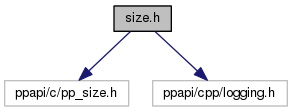Pepper_42_C++_interfaces
size.h File Reference
`#include "ppapi/c/pp_size.h"`
`#include "ppapi/cpp/logging.h"`
Include dependency graph for size.h:Go to the source code of this file.

## Classes

class  pp::Size
A size of an object based on width and height. More...

class  pp::FloatSize
A size of an object based on width and height. More...

## Namespaces

pp
The C++ interface to the Pepper API.

## Functions

bool operator== (const pp::Size &lhs, const pp::Size &rhs)

bool operator!= (const pp::Size &lhs, const pp::Size &rhs)

bool operator== (const pp::FloatSize &lhs, const pp::FloatSize &rhs)

bool operator!= (const pp::FloatSize &lhs, const pp::FloatSize &rhs)

## Detailed Description

This file defines the API to create a size based on width and height.

Definition in file size.h.

## Function Documentation

 bool operator!= ( const pp::Size & lhs, const pp::Size & rhs )
inline

This function determines whether two `Sizes` are not equal.

Parameters
 [in] lhs The `Size` on the left-hand side of the equation. [in] rhs The `Size` on the right-hand side of the equation.
Returns
true if the `Size` of lhs are equal to the `Size` of rhs, otherwise false.

Definition at line 303 of file size.h.

 bool operator!= ( const pp::FloatSize & lhs, const pp::FloatSize & rhs )
inline

This function determines whether two `FloatSizes` are not equal.

Parameters
 [in] lhs The `FloatSize` on the left-hand side of the equation. [in] rhs The `FloatSize` on the right-hand side of the equation.
Returns
true if the `FloatSize` of lhs are equal to the `FloatSize` of rhs, otherwise false.

Definition at line 329 of file size.h.

 bool operator== ( const pp::Size & lhs, const pp::Size & rhs )
inline

This function determines whether the width and height values of two sizes are equal.

Parameters
 [in] lhs The `Size` on the left-hand side of the equation. [in] rhs The `Size` on the right-hand side of the equation.
Returns
true if they are equal, false if unequal.

Definition at line 292 of file size.h.

References pp::Size::height(), and pp::Size::width().

 bool operator== ( const pp::FloatSize & lhs, const pp::FloatSize & rhs )
inline

This function determines whether the width and height values of two sizes are equal.

Parameters
 [in] lhs The `FloatSize` on the left-hand side of the equation. [in] rhs The `FloatSize` on the right-hand side of the equation.
Returns
true if they are equal, false if unequal.

Definition at line 316 of file size.h.

References pp::FloatSize::height(), and pp::FloatSize::width().